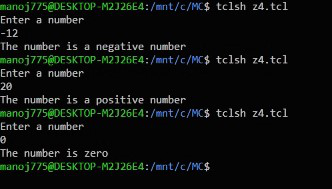Open in App
Not now

# TCL script to determine whether a number is positive, negative or zero using if-else statement

• Last Updated : 26 Apr, 2021

In this article, we will know the syntax of the if-else statement in Tool Command Language. We will understand the TCL script to determine whether a number is positive, negative, or zero using an if-else statement with the help of an example. Let’s discuss it one by one.

Pre-requisite –
https://www.geeksforgeeks.org/basics-of-ns2-and-otcltcl-script/.

Overview :
To understand it better we will go through an example that covers all of its variations. To further understand this statement, we will compare its syntax with that of the C language.

Example –
Let’s take a simple example where we wish to find whether a number is positive, negative, or zero. Let’s understand this code in the following steps as follows.

Step-1 :
Our first step is to read the input number.  We prompt the user and get the input using gets.

```puts "Enter a number"
gets stdin number```

Step-2 :
Our next step is to appropriate if-else condition mapping. This can be done as follows.

```if {\$number>0} {
puts "The number is a positive number"
} elseif {\$number<0} {
puts "The number is a negative number"
} else {
puts "The number is zero"
}```

Note –
The syntax of the if-else must be exactly as shown above. If you neglect the spaces or type the opening curly brace in a new line, the result will be an error.

Step-3 :
Now let’s compare the above piece of code to what it would look like in C language to understand the syntax better as follows.

```if(number>0)
{
printf("The number is a positive number");
} else if(number<0)
{
printf("The number is a negative number");
}
else
{
printf("The number is zero");
}```

Step-4 :
The whole code with the output is as follows.

Code –

```puts "Enter a number "
gets stdin number
if {\$number>0} {
puts "The number is a positive number"
} elseif {\$number<0} {
puts "The number is a negative number"
} else {
puts "The number is zero"
}```

Output :My Personal Notes arrow_drop_up